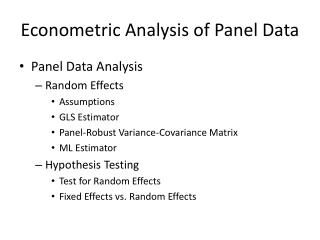DownloadDownload PresentationEconometric Analysis of Panel Data

# Econometric Analysis of Panel Data

Télécharger la présentation## Econometric Analysis of Panel Data

- - - - - - - - - - - - - - - - - - - - - - - - - - - E N D - - - - - - - - - - - - - - - - - - - - - - - - - - -
##### Presentation Transcript

1. Econometric Analysis of Panel Data • Panel Data Analysis • Random Effects • Assumptions • GLS Estimator • Panel-Robust Variance-Covariance Matrix • ML Estimator • Hypothesis Testing • Test for Random Effects • Fixed Effects vs. Random Effects

2. Panel Data Analysis • Random Effects Model • ui is random, independent of eit and xit. • Define eit = ui + eit the error components.

3. Random Effects Model • Assumptions • Strict Exogeneity • X includes a constant term, otherwise E(ui|X)=u. • Homoschedasticity • Constant Auto-covariance (within panels)

4. Random Effects Model • Assumptions • Cross Section Independence

5. Random Effects Model • Extensions • Weak Exogeneity • Heteroscedasticity

6. Random Effects Model • Extensions • Serial Correlation • Spatial Correlation

7. Model Estimation: GLS • Model Representation

8. Model Estimation: RE-OLS • Partial Group Mean Deviations

9. Model Estimation: RE-OLS • Model Assumptions • OLS

10. Model Estimation: RE-OLS • Need a consistent estimator of q: • Estimate the fixed effects model to obtain • Estimate the between model to obtain • Or, estimate the pooled model to obtain • Based on the estimated large sample variances, it is safe to obtain

11. Model Estimation: RE-OLS • Panel-Robust Variance-Covariance Matrix • Consistent statistical inference for general heteroscedasticity, time series and cross section correlation.

12. Model Estimation: ML • Log-Likelihood Function

13. Model Estimation: ML • ML Estimator

14. Hypothesis TestingTo Pool or Not To Pool, Continued • Test for Var(ui) = 0, that is • If Ti=T for all i, the Lagrange-multiplier test statistic (Breusch-Pagan, 1980) is:

15. Hypothesis TestingTo Pool or Not To Pool, Continued • For unbalanced panels, the modified Breusch-Pagan LM test for random effects (Baltagi-Li, 1990) is: • Alternative one-side test:

16. Hypothesis TestingTo Pool or Not To Pool, Continued • References • Baltagi, B. H., and Q. Li, A Langrange Multiplier Test for the Error Components Model with Incomplete Panels, Econometric Review, 9, 1990, 103-107. • Breusch, T. and A. Pagan, “The LM Test and Its Applications to Model Specification in Econometrics,” Review of Economic Studies, 47, 1980, 239-254.

17. Hypothesis TestingFixed Effects vs. Random Effects

18. Hypothesis TestingFixed Effects vs. Random Effects • Fixed effects estimator is consistent under H0 and H1; Random effects estimator is efficient under H0, but it is inconsistent under H1. • Hausman Test Statistic

19. Hypothesis TestingFixed Effects vs. Random Effects • Alternative (Asym. Eq.) Hausman Test • Estimate any of the random effects models • F Test that g = 0

20. Hypothesis TestingFixed Effects vs. Random Effects • Ahn-Low Test (1996) • Based on the estimated errors (GLS residuals) of the random effects model, estimate the following regression:

21. Hypothesis TestingFixed Effects vs. Random Effects • References • Ahn, S.C., and S. Low, A Reformulation of the Hausman Test for Regression Models with Pooled Cross-Section Time-Series Data, Journal of Econometrics, 71, 1996, 309-319. • Baltagi, B.H., and L. Liu, Alternative Ways of Obtaining Hausman’s Test Using Artificial Regressions, Statistics and Probability Letters, 77, 2007, 1413-1417. • Hausman, J.A., Specification Tests in Econometrics, Econometrica, 46, 1978, 1251-1271. • Hausman, J.A. and W.E. Taylor, Panel Data and Unobservable Individual Effects, Econometrics, 49, 1981, 1377-1398. • Mundlak, Y., On the Pooling of Time Series and Cross-Section Data, Econometrica, 46, 1978, 69-85.

22. Example: Investment Demand • Grunfeld and Griliches  • i = 10 firms: GM, CH, GE, WE, US, AF, DM, GY, UN, IBM; t = 20 years: 1935-1954 • Iit = Gross investment • Fit = Market value • Cit = Value of the stock of plant and equipment